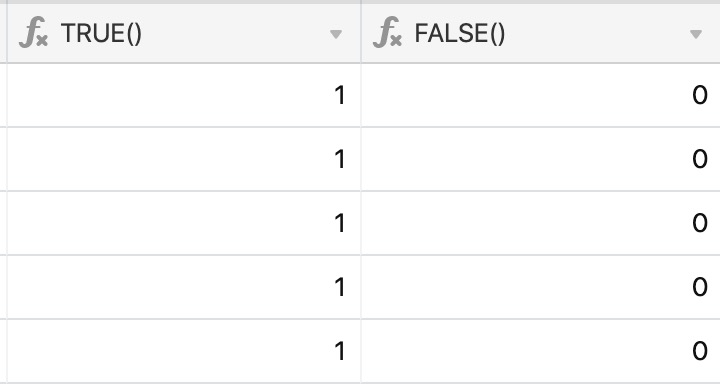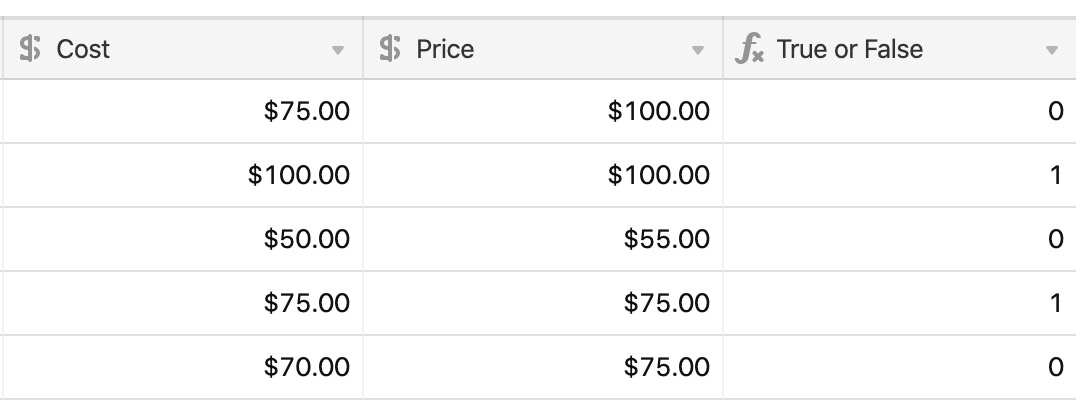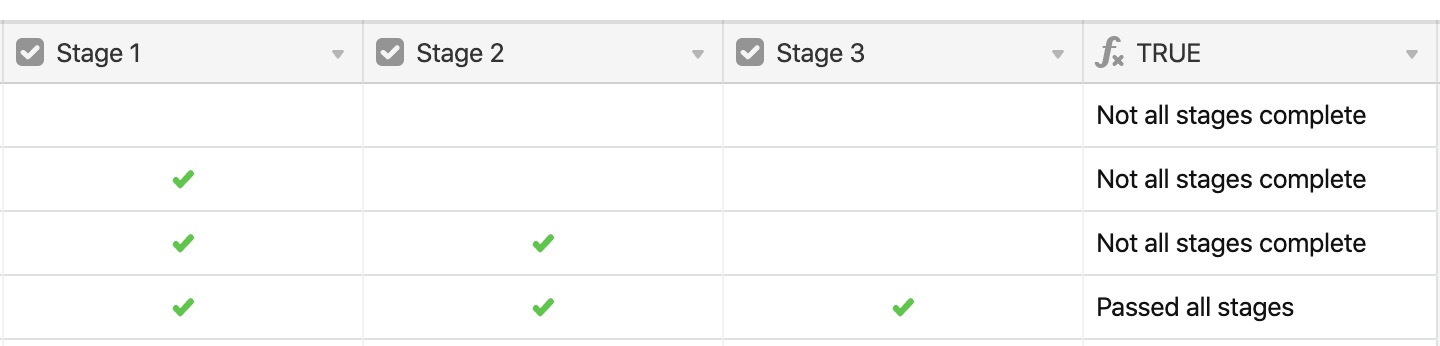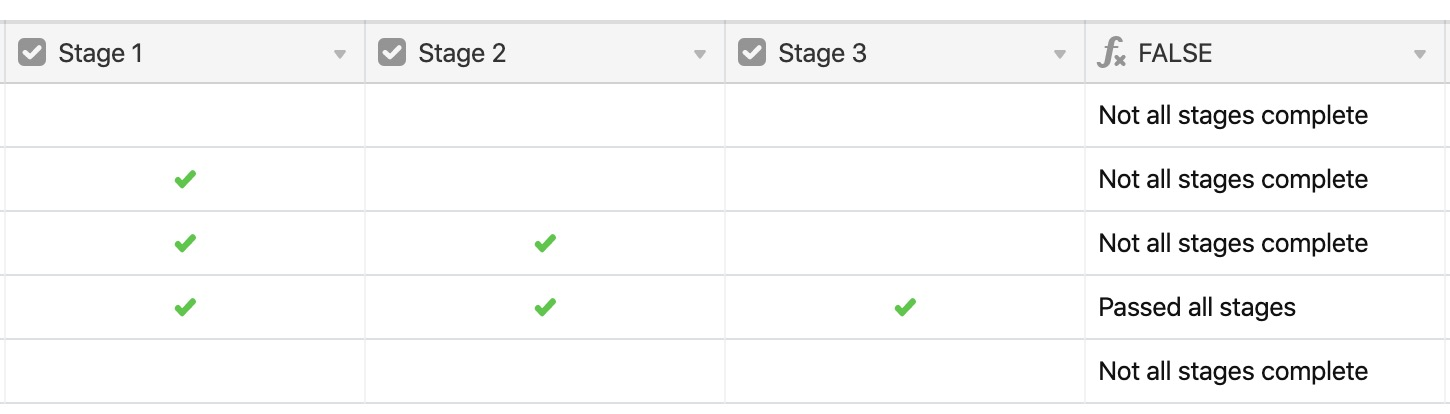• English
• Account
• API
• Integrations
• Enterprise
• Resources
Using true and false functions in Airtable
• 21 Nov 2022
• Dark
Light
• PDF

# Using true and false functions in Airtable

• Dark
Light
• PDF

Article Summary

When creating conditional formulas, `TRUE()`and `FALSE() `functions assist in yielding specific true and false results when you need to explicitly provide that type of output. This article discusses how these functions work and common ways to use them in your formulas. This article is part of a guided course which you can view here.

The `TRUE()`and `FALSE() `functions are very straightforward in their use. They each generate the logical value true and false, respectively, and output a `1 `or a `0`.These functions are more commonly used within a conditional statement, like this:

``````IF ({Cost} = {Price}, TRUE (), FALSE ())
``````## True

Using another example scenario, let's say that you're a hiring manager and want to track candidates who have made it through three major stages in the hiring process.

You could use `TRUE() `within a conditional statement to output one message if they passed all stages, and another message if they didn't.

``````IF(
AND(
{Stage 1}=TRUE(),
{Stage 2}=TRUE(),
{Stage 3}=TRUE()
),
"Passed all stages",
"Not all stages complete"
)``````

## False

Let's use the same hiring process scenario as before, except we'll structure the formula differently to utilize `FALSE()`instead.

``````IF(
AND(
{Stage 1},
{Stage 2},
{Stage 3}
)
=
FALSE(),
"Not all stages complete",
"Passed all stages"
)``````

You'll notice that the two different formulas output the exact same result (see examples below).``````IF(
AND(
{Stage 1}=TRUE(),
{Stage 2}=TRUE(),
{Stage 3}=TRUE()
),
"Passed all stages",
"Not all stages complete"
)````````````IF(
AND(
{Stage 1},
{Stage 2},
{Stage 3}
)
=
FALSE(),
"Not all stages complete",
"Passed all stages"
)``````